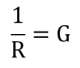An electrical conductivity meter is a device for determining the electrical resistance R of a solution located between two platinum plates of a conductivity cell. An alternating voltage (100-1000Hz) of low amplitude (to avoid electrolysis and electrode polarization) is applied between the two plates. Resistance is then determined thanks to a complex electrical circuit (Wheatstone bridge). Conductance is defined as the inverse of the resistance R and is noted as G:The conductivity noted σ is proportional to G with k a proportionality factor called cell constant which depends on the conductivity cell used:

σ = kG

where k = I/S (IUPAC recommendation) is the cell constant related to the physical characteristics of the conductivity cell (I the distance between the two plates and S the actual surface area of the two plates).For a new conductivity cell, the value for k given by the manufacturer is often close to the unit (k = 1).Next page
Previous page